# F.E.A.R. 3 Walkthrough Interval 01: Prison

September 12, 2016 (Last Updated: ) | Reading Time: 4 minutes

This F.E.A.R. 3 walkthrough is divided into 8 total pages.

1 - Interval 01: Prison

You can jump to nearby pages of the game using the links above.

## Interval 01: Prison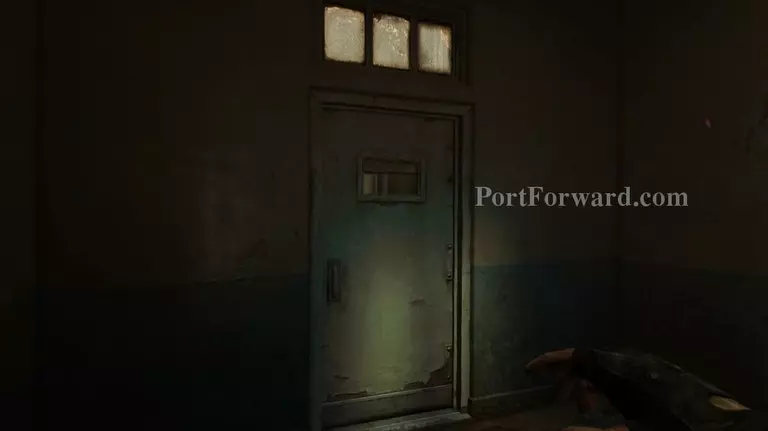F.E.A.R. 3 Walkthrough - F.E.A.R. 3 0

Get out of the room and follow the corridor.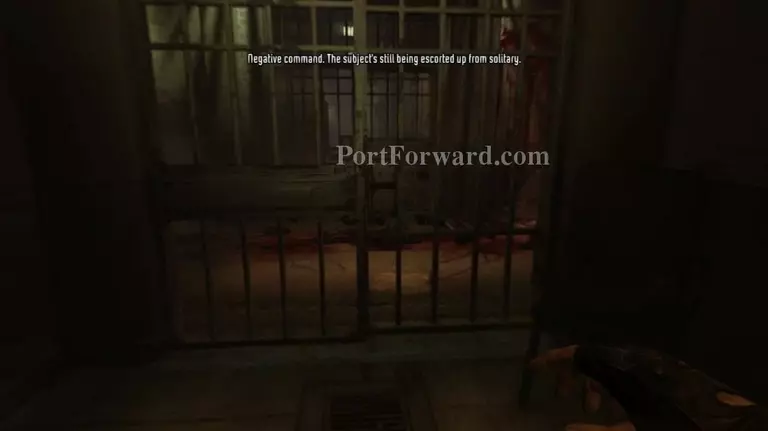F.E.A.R. 3 Walkthrough - F.E.A.R. 3 1

Open the gate and continue going to the left.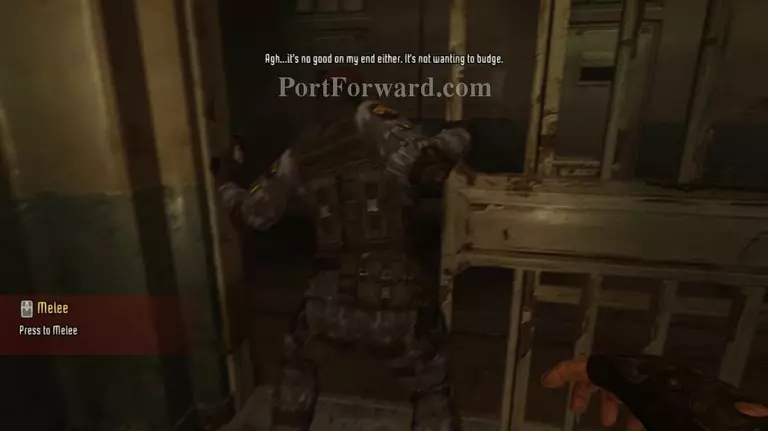F.E.A.R. 3 Walkthrough - F.E.A.R. 3 2

Stab the guard and take his gun.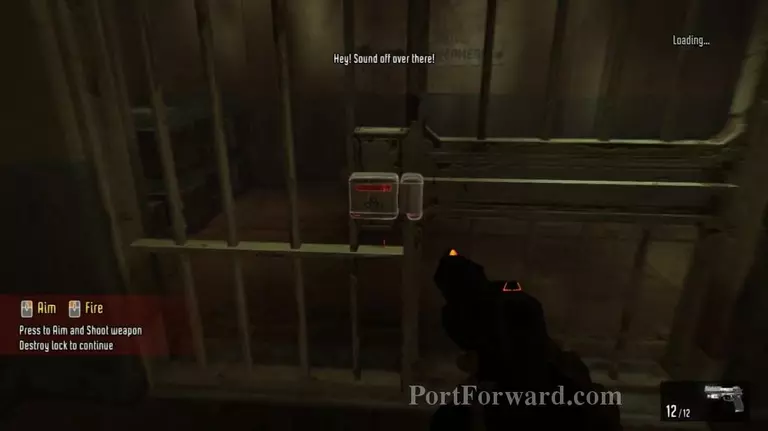F.E.A.R. 3 Walkthrough - F.E.A.R. 3 3

To the left there'll be a locked gate. You can shoot or stab the lock to get it open.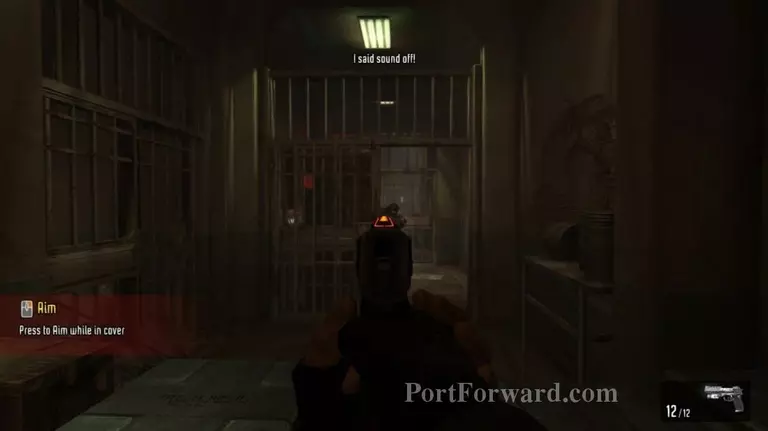F.E.A.R. 3 Walkthrough - F.E.A.R. 3 4

Get rid of the guard ahead.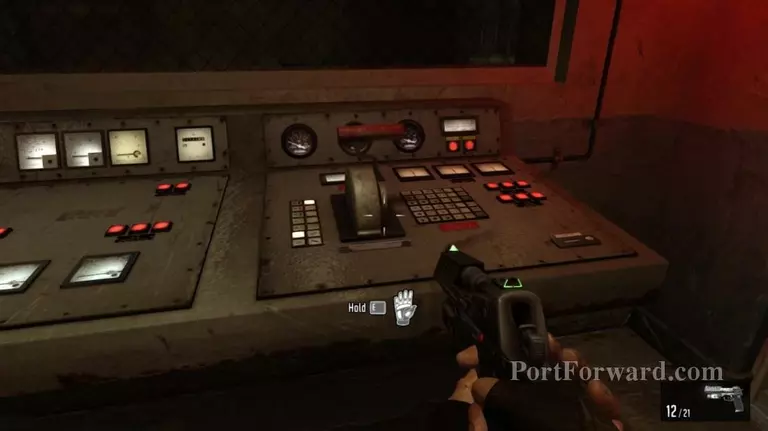F.E.A.R. 3 Walkthrough - F.E.A.R. 3 5

Pull the lever to open the gate.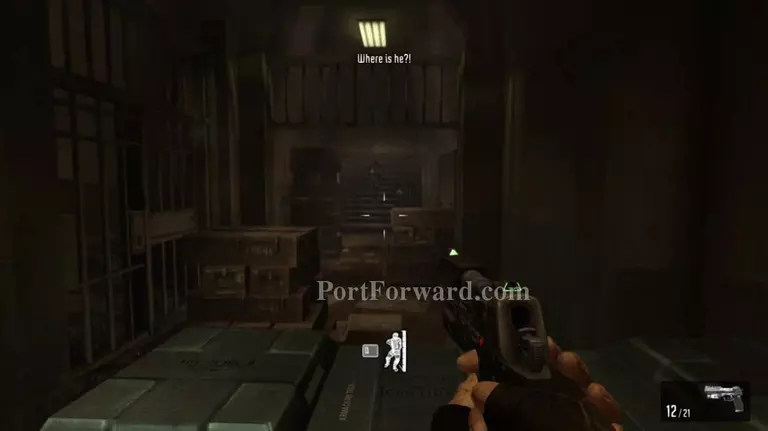F.E.A.R. 3 Walkthrough - F.E.A.R. 3 6

More guards will appear. Take them out and continue forward.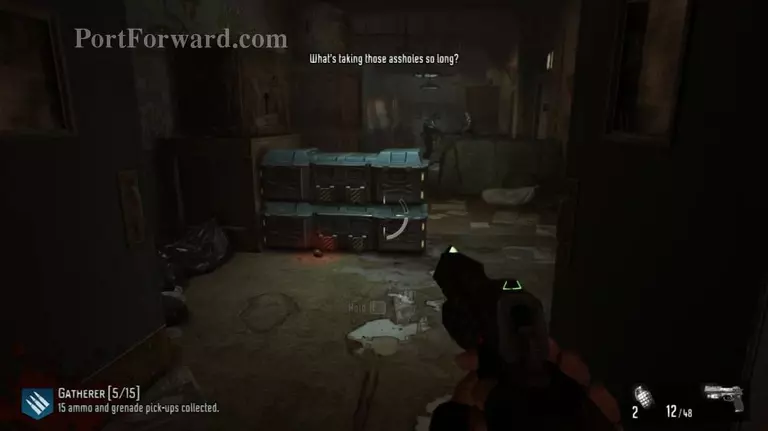F.E.A.R. 3 Walkthrough - F.E.A.R. 3 7

Up the stairs you'll find more guards. Kill them all then go through the door at the end of the corridor.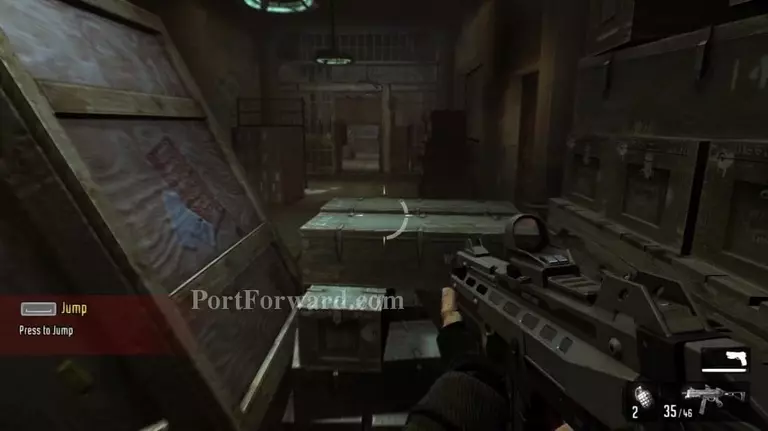F.E.A.R. 3 Walkthrough - F.E.A.R. 3 8

Jump over the crates.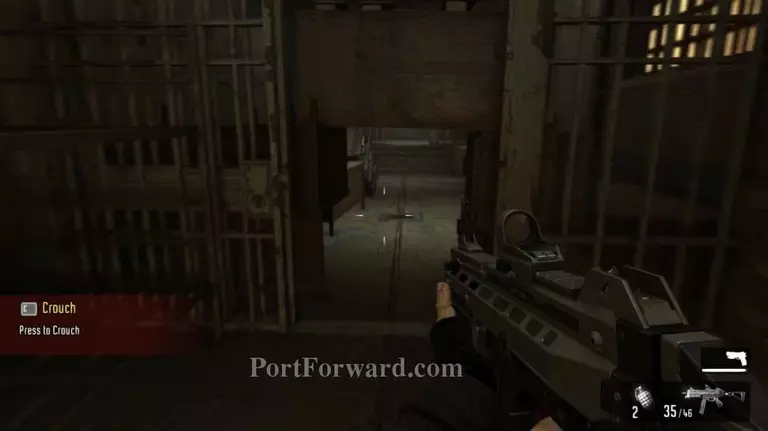F.E.A.R. 3 Walkthrough - F.E.A.R. 3 9

Crouch past the obstacles.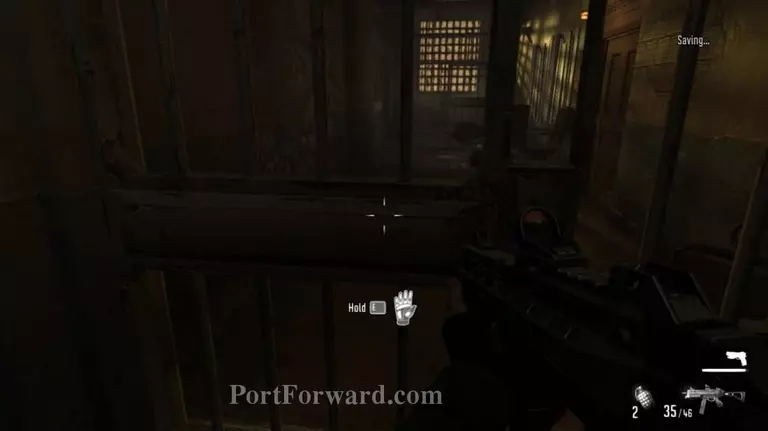F.E.A.R. 3 Walkthrough - F.E.A.R. 3 10

Open the gate on the left then in through the first door on the left. You can kick open doors with the melee key.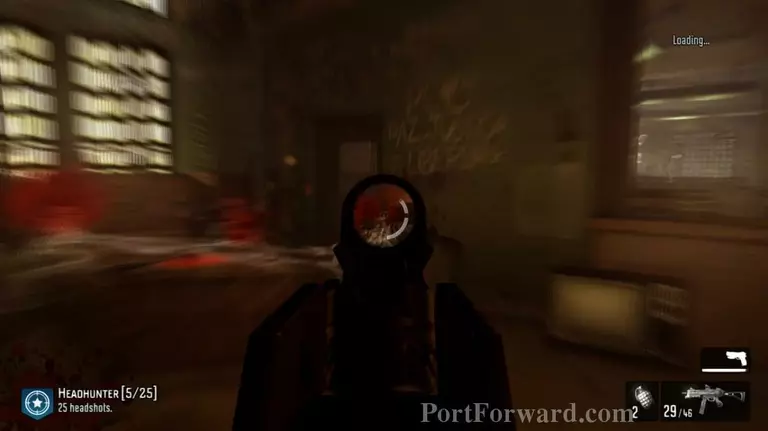F.E.A.R. 3 Walkthrough - F.E.A.R. 3 11

The place will be filled with guards. Make your way to the other end of the area.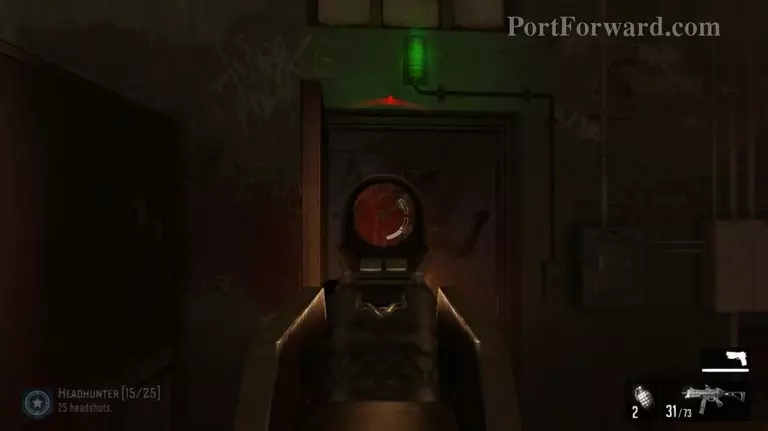F.E.A.R. 3 Walkthrough - F.E.A.R. 3 12

Through the door with the green light on top.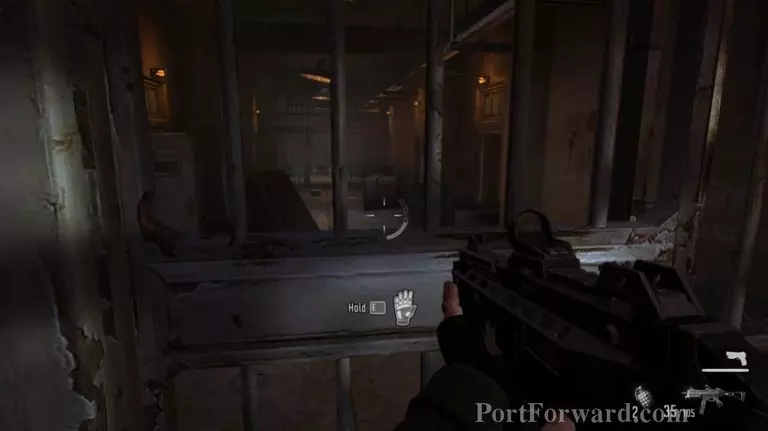F.E.A.R. 3 Walkthrough - F.E.A.R. 3 13

Open the gate, get to the end of the hallway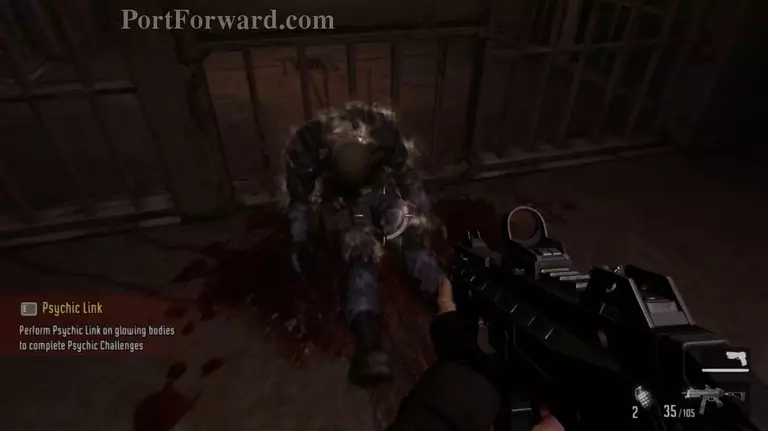F.E.A.R. 3 Walkthrough - F.E.A.R. 3 14

Hold E in front of the body, then open the gate and go through.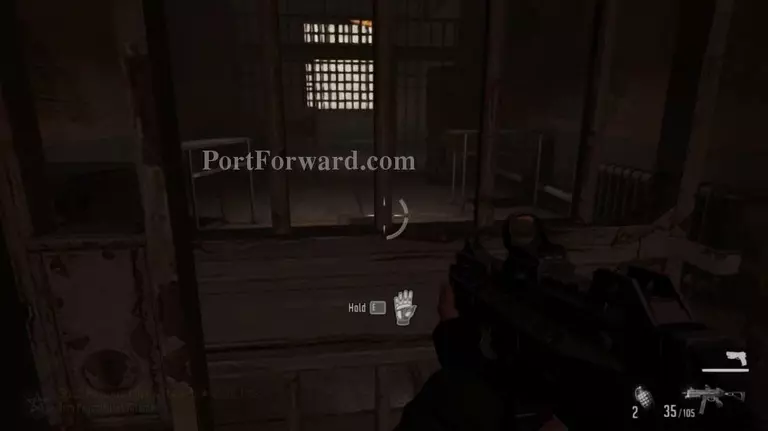F.E.A.R. 3 Walkthrough - F.E.A.R. 3 15

Open the next gate and keep going.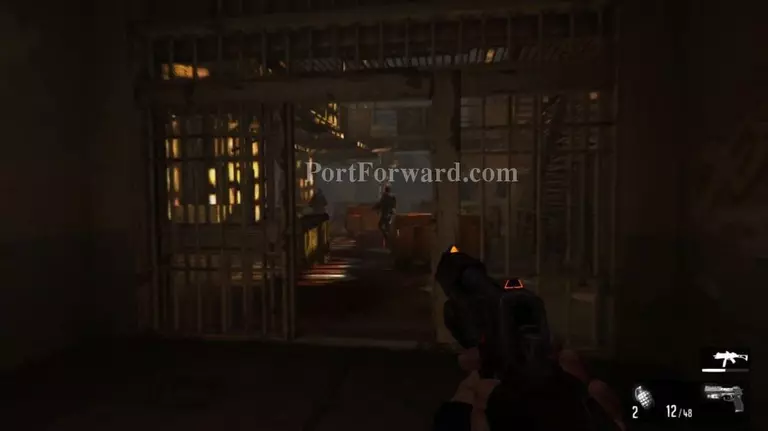F.E.A.R. 3 Walkthrough - F.E.A.R. 3 16

There'll be plenty of thugs ahead.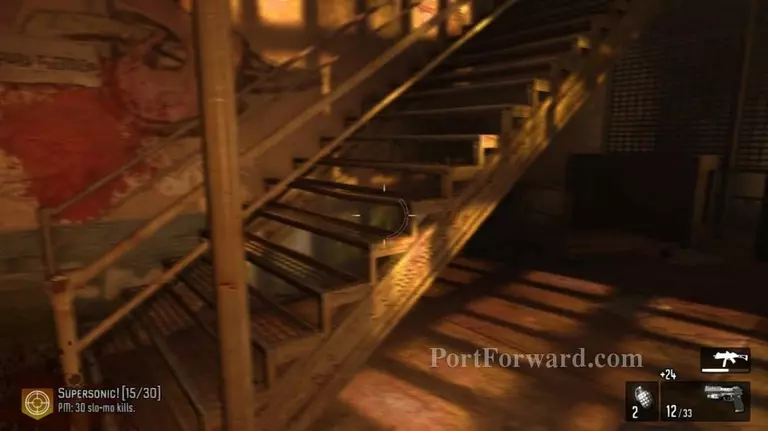F.E.A.R. 3 Walkthrough - F.E.A.R. 3 17

Stick to the left hand wall and you'll find a staircase. Climb up and be ready to fight some more guards.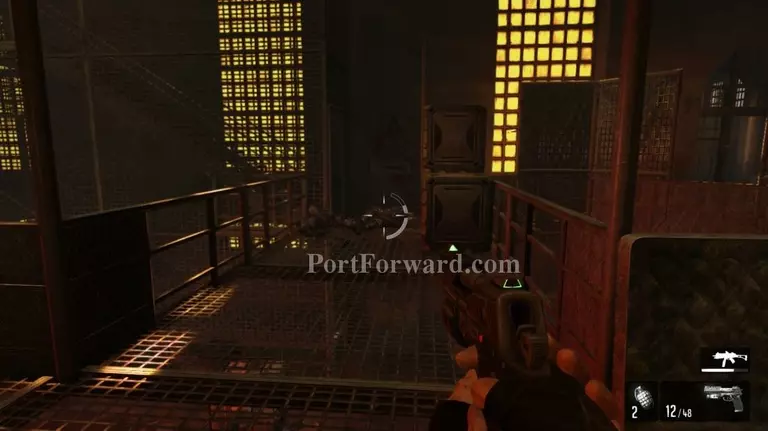F.E.A.R. 3 Walkthrough - F.E.A.R. 3 18

Go across the walkway to the other side.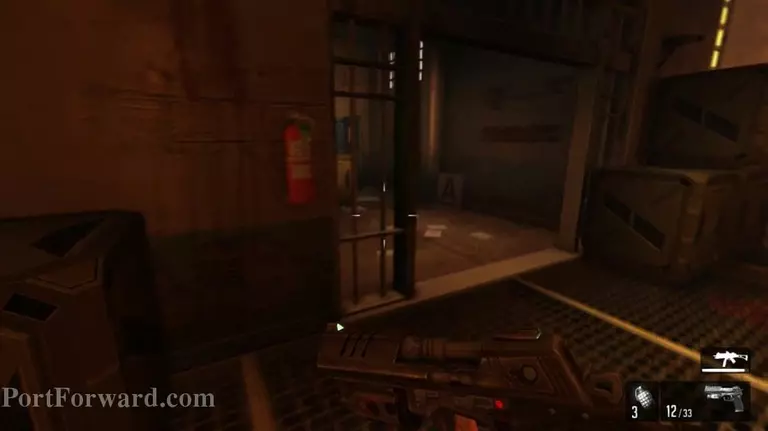F.E.A.R. 3 Walkthrough - F.E.A.R. 3 19

Through the path on the left and down the stairs.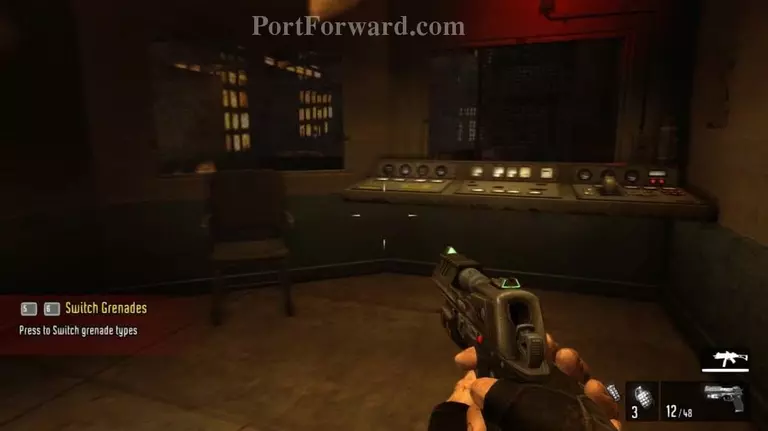F.E.A.R. 3 Walkthrough - F.E.A.R. 3 20

Pull the lever on the right to open the gate.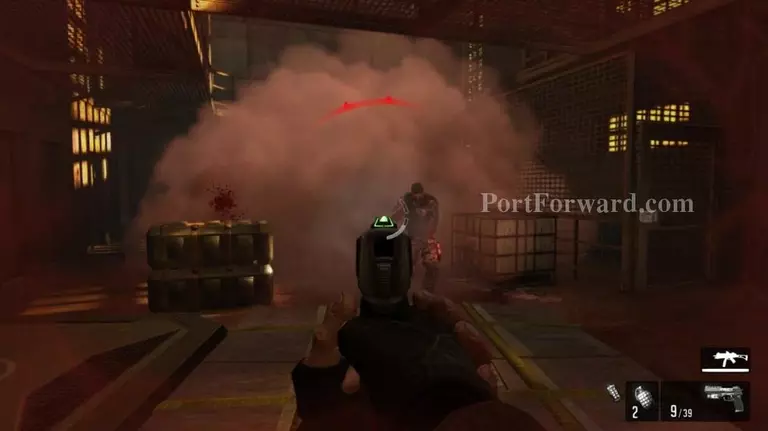F.E.A.R. 3 Walkthrough - F.E.A.R. 3 21

Enemies will appear. Take them out then continue through the gate behind them.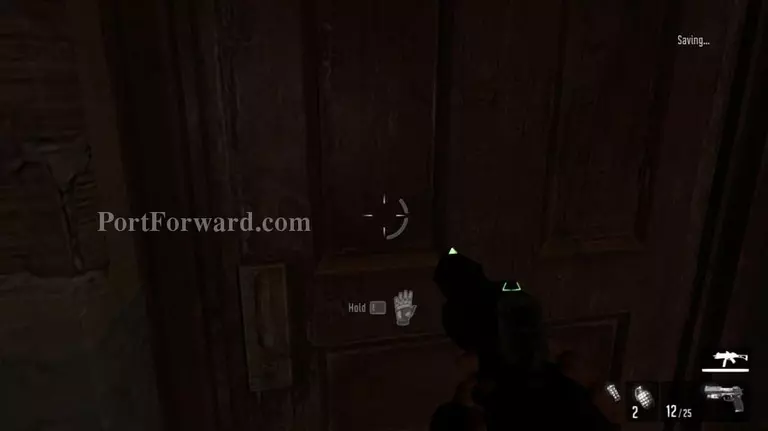F.E.A.R. 3 Walkthrough - F.E.A.R. 3 22

Enter the room to the left. More guards ahead.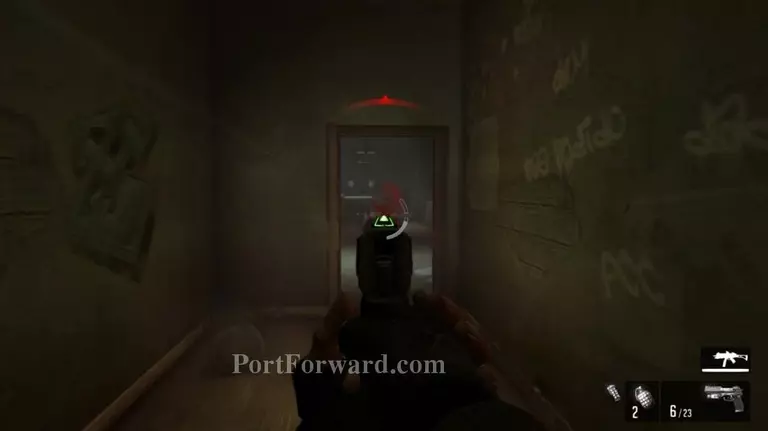F.E.A.R. 3 Walkthrough - F.E.A.R. 3 23

Go through the door at the other side of the room then keep going to the end of the hall.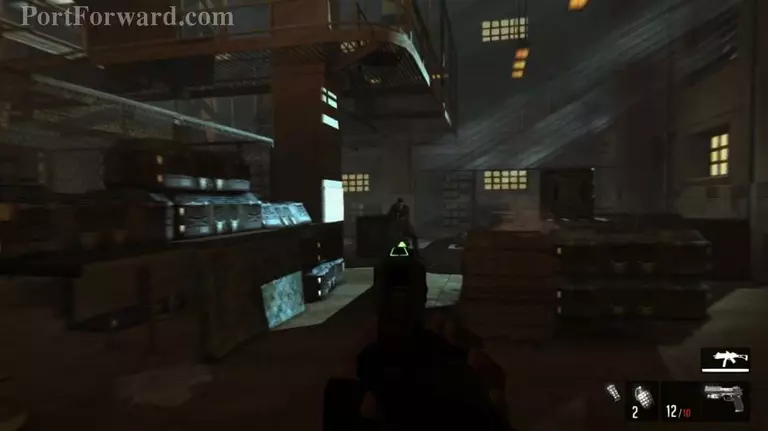F.E.A.R. 3 Walkthrough - F.E.A.R. 3 24

Plenty of guards will be ahead.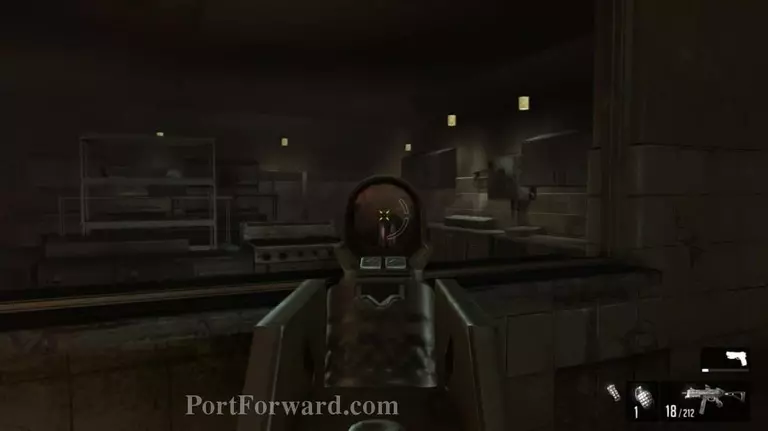F.E.A.R. 3 Walkthrough - F.E.A.R. 3 25

Finish off the enemies in the kitchen then go inside.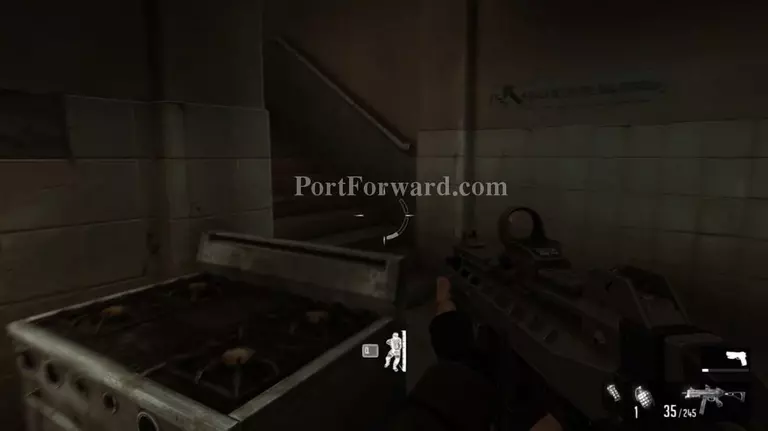F.E.A.R. 3 Walkthrough - F.E.A.R. 3 26

Walk up the stairs.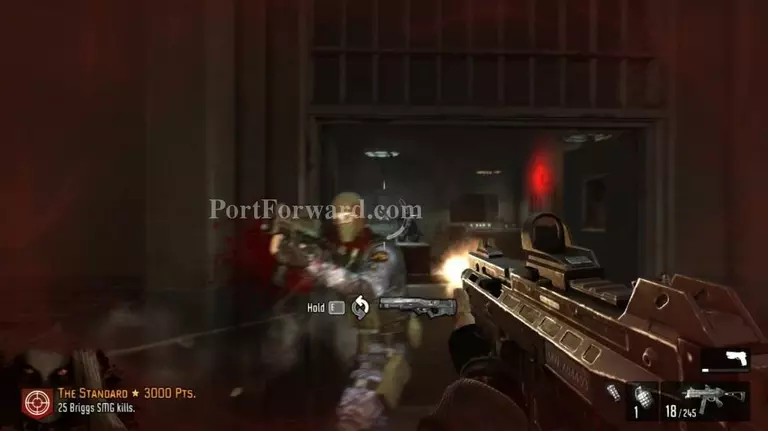F.E.A.R. 3 Walkthrough - F.E.A.R. 3 27

Kill the remaining guards.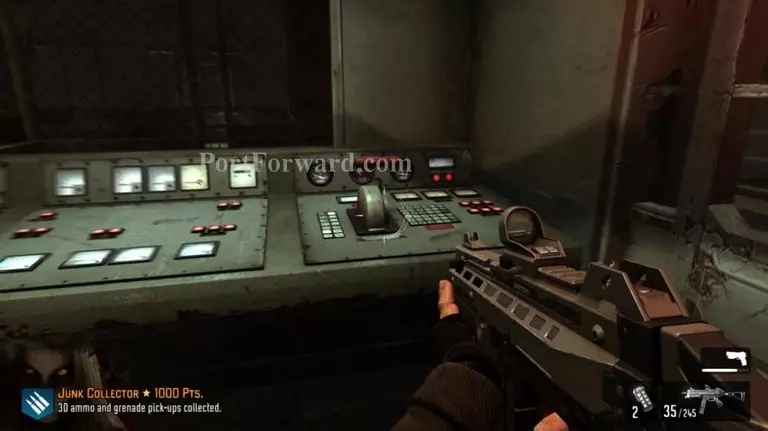F.E.A.R. 3 Walkthrough - F.E.A.R. 3 28

Pull the lever to open the gate beside you. It may take longer to open than the previous gates.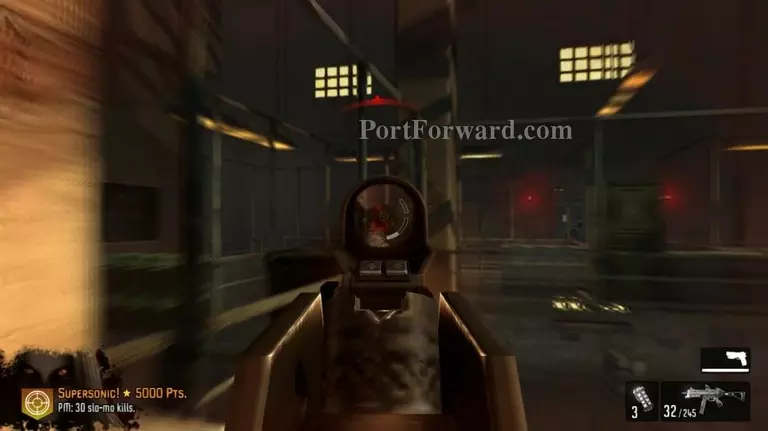F.E.A.R. 3 Walkthrough - F.E.A.R. 3 29

Shoot all the enemies on the walkways.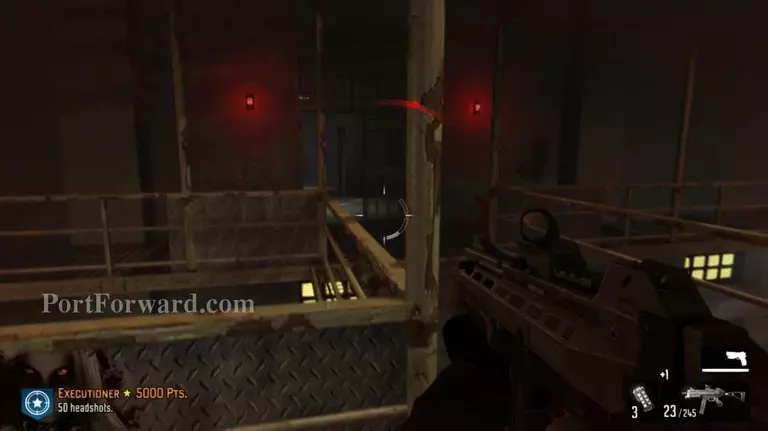F.E.A.R. 3 Walkthrough - F.E.A.R. 3 30

Go into the gate with the red lights at the sides.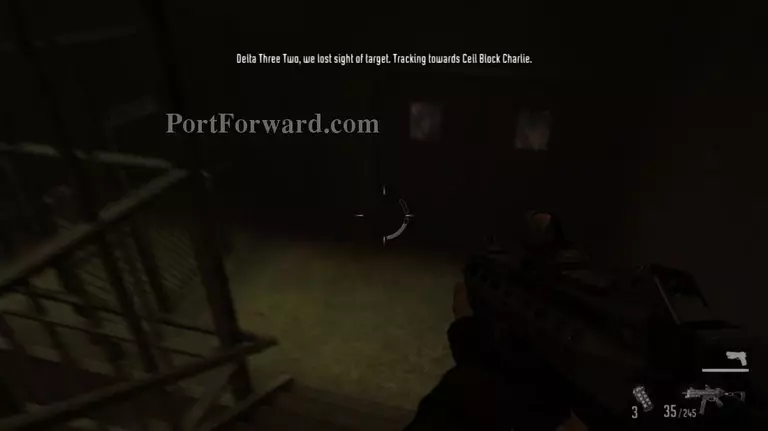F.E.A.R. 3 Walkthrough - F.E.A.R. 3 31

Down the stairs, through the door at the bottom.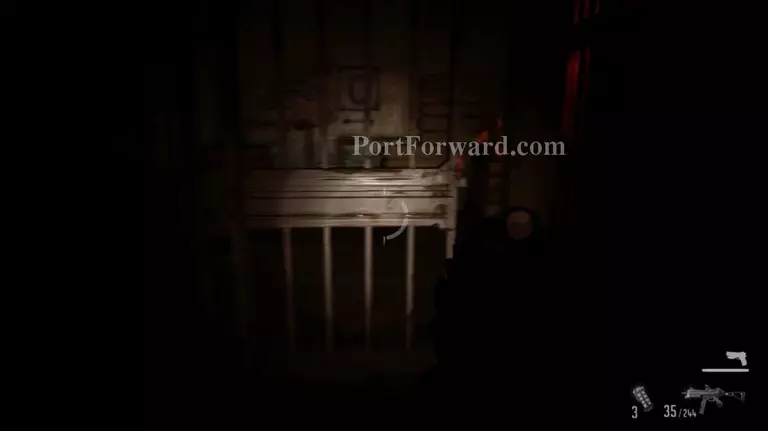F.E.A.R. 3 Walkthrough - F.E.A.R. 3 32

Go through the first gate then through the second and third one on the right.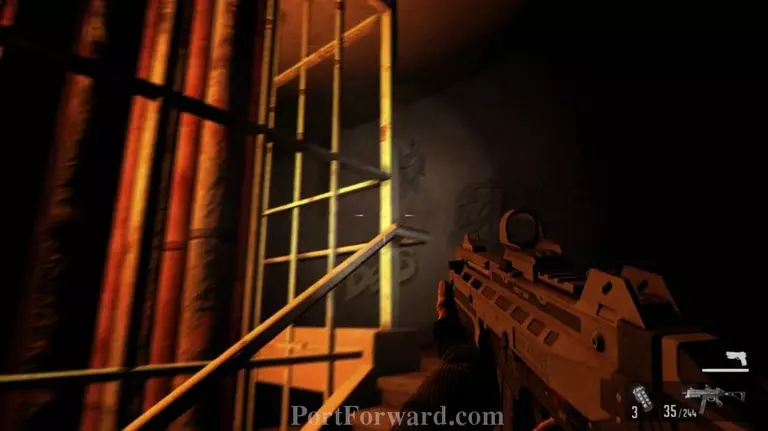F.E.A.R. 3 Walkthrough - F.E.A.R. 3 33

Walk up the stairs. Crouch to avoid the flames.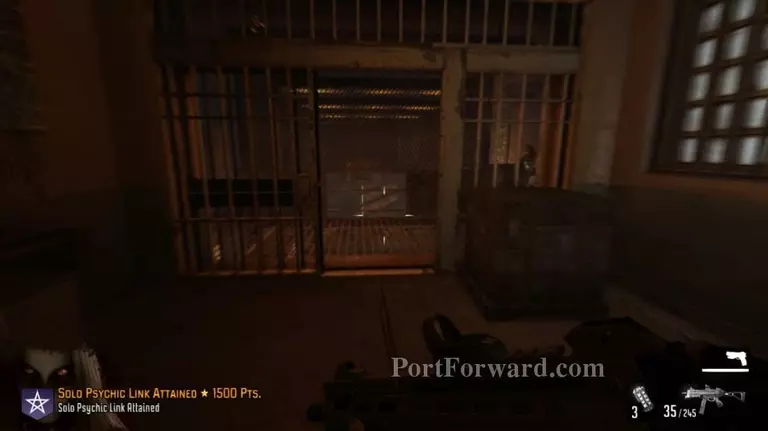F.E.A.R. 3 Walkthrough - F.E.A.R. 3 34

You'll come up to another walkway full of enemies. Make your way forward to the far side of the area.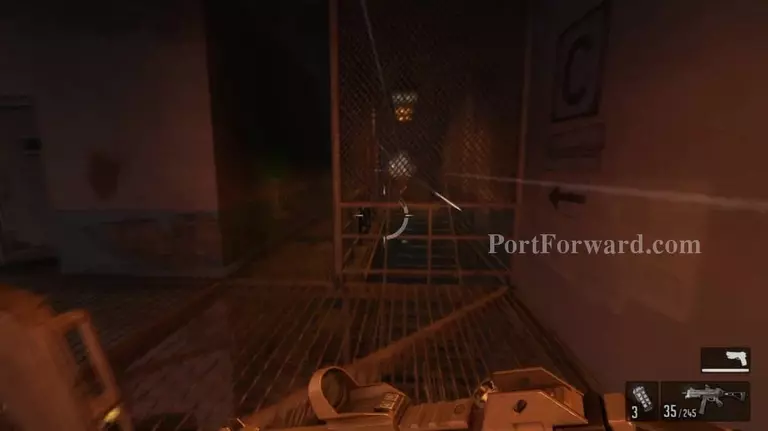F.E.A.R. 3 Walkthrough - F.E.A.R. 3 35

Go down the stairs.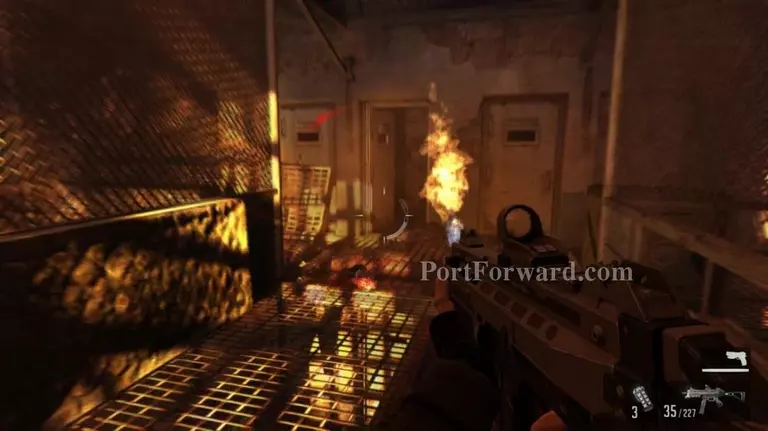F.E.A.R. 3 Walkthrough - F.E.A.R. 3 36

You'll see an open cell door. Go inside.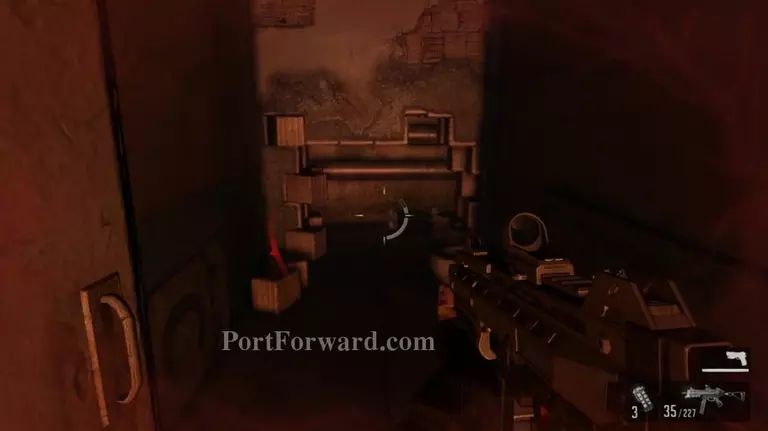F.E.A.R. 3 Walkthrough - F.E.A.R. 3 37

Crouch into the hole on the wall.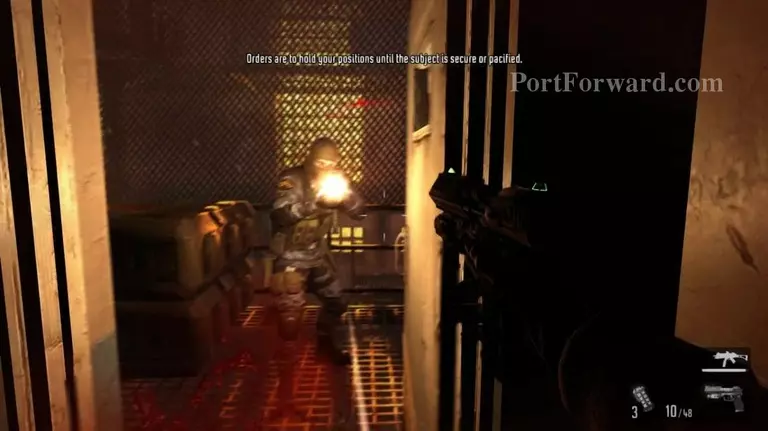F.E.A.R. 3 Walkthrough - F.E.A.R. 3 38

You'll find more guards outside the cell.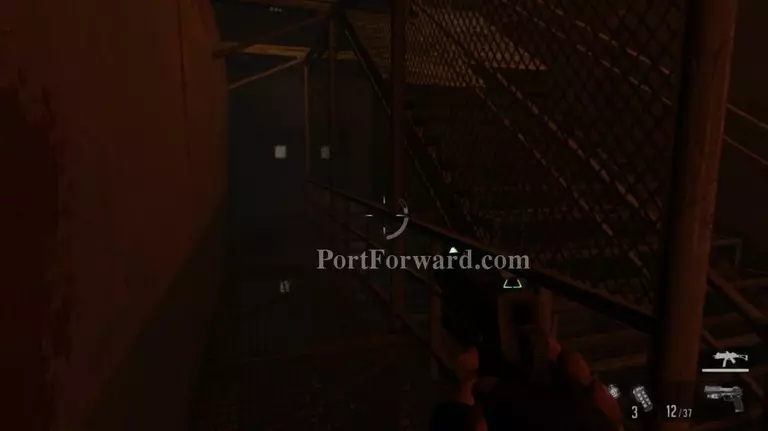F.E.A.R. 3 Walkthrough - F.E.A.R. 3 39

Take the stairs down, to the right.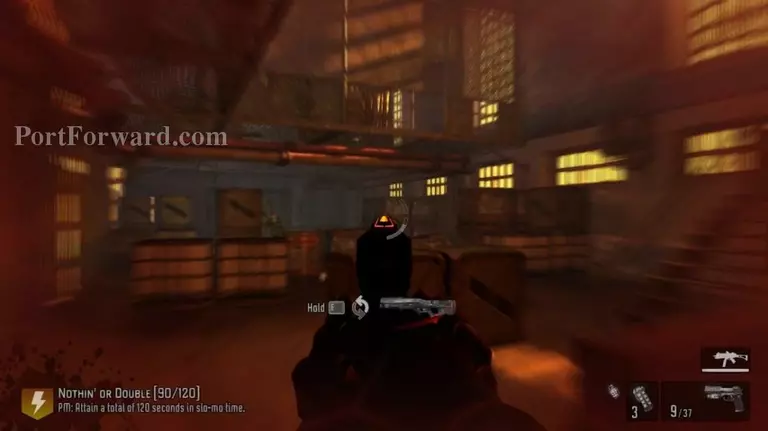F.E.A.R. 3 Walkthrough - F.E.A.R. 3 40

Eliminate all the remaining enemies.

## More F.E.A.R. 3 Walkthroughs

This F.E.A.R. 3 walkthrough is divided into 8 total pages.

1 - Interval 01: Prison